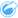# Create Regression Model

Create Regression Model is used to model the relationship between two or more explanatory variables and a response variable by fitting a linear equation to observed data. Every value of the independent variable (x) is associated with a value of the dependent variable (y).

Create Regression Model uses Ordinary Least Squares (OLS) as the regression type.

## Example

An environmental organization is studying the cause of greenhouse gas emissions by country from 1990 to 2015. Create Regression Model can be used to create an equation that can estimate the amount of greenhouse gas emissions per country based on explanatory variables such as population and gross domestic product (GDP).

## Use the Create Regression Model capability

Use the following steps to run the Create Regression Model analysis capability:

1. Create a map, chart, or table using the dataset with which you want to create a regression model.
2. Click the Action button.
3. Do one of the following:
• If your card is a chart or table, click How is it related in the Analytics pane.
• If your card is a map, click the Find answers tab and click How is it related.
4. Click Create Regression Model.
5. For Choose a layer, select the dataset with which you want to create a regression model.
6. For Choose a dependent variable, choose the field you want to explain with your model. The field must be a number or rate/ratio.
7. Click Select explanatory variables to display a menu of available fields.
8. Select the fields to use as explanatory variables (also called independent variables).
9. Click Select to apply the explanatory variables.
10. Click the Visualize button to view a scatter plot or scatter plot matrix of the dependent and explanatory variables, if available. The scatter plots can be used as part of the exploratory analysis for your model.
##### Note:

The Visualize button is unavailable if five or more explanatory variables are chosen.

11. Click Run.

The regression model is created for your chosen dependent and explanatory variables. You can now use the outputs and statistics to continue verifying the model validity with exploratory and confirmatory analysis.

## Usage notes

Create Regression Model can be found using the Action buttonunder How is it related on the Find answers tab.

One number or rate/ratio field can be chosen as the dependent variable. The dependent variable is the number field that you are trying to explain with your regression model. For example, if you are creating a regression model to determine the causes of child mortality, the child mortality rate is the dependent variable.

Up to 20 number or rate/ratio fields can be chosen as explanatory variables. Explanatory variables are independent variables that can be chosen as part of the regression model to explain the dependent variable. For example, if you are creating a regression model to determine the causes of child mortality, explanatory variables may include poverty rates, disease rates, and vaccination rates. If the number of explanatory variables chosen is four or fewer, a scatter plot or scatter plot matrix can be created by clicking Visualize.

The following output values are given under Model statistics:

• Regression equation
• R2
• Durbin-Watson test
• p-value
• Residual standard error
• F statistic

The outputs and statistics can be used to analyze the accuracy of the model.

After you create the model, a new function dataset is added to the data pane. The function dataset can be used in the Predict Variable capability. Create Regression Model also creates a result dataset that includes all the fields from the input plus estimated, residual, and standardized_residual fields. The fields contain the following information:

• estimated—The value of the dependent variable as estimated by the regression model
• residual—The difference between the original field value and the estimated value of the dependent variable
• standardized_residual—The ratio of the residual and the standard deviation of the residual

## How Create Regression Model works

An Ordinary Least Squares model can be created if the following assumptions are met:

• The model must be linear in the parameters.
• The data is a random sample of the population.
• The independent variables are not too strongly collinear.
• The independent variables are measured precisely such that measurement error is negligible.
• The expected value of the residuals is always zero.
• The residuals have constant variance (homogeneous variance).
• The residuals are normally distributed.

Create Regression Model often runs successfully even if one or more of the assumptions are not met. Therefore, the assumptions for OLS should be tested before using Create Regression Model. If the assumptions are not met, the model may not be valid.

A model cannot be created if the third assumption—the independent variables are not too strongly collinear—is not met. In that case, the message Two or more explanatory variables are related. Remove one of the collinear variables and try again appears. You can determine which variables are collinear using a scatter plot or scatter plot matrix. The collinear variables will have a linear relationship and one of the variables will have a strong dependency on the other. Remove the dependent collinear variable from the model.

For more information on the assumptions of OLS models, see Regression analysis.